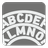Vigenere Cipher
Elementary+
English FR RU

The Vigenère cipher is a method of encrypting alphabetic text by using a series of different Caesar ciphers based on the letters of a keyword. It is a simple form of polyalphabetic substitution.

In the Vigenère cipher each letter of a message is shifted along some number of places with different shift values. To encrypt, a table of alphabets can be used, termed a tabula recta, Vigenère square, or Vigenère table. It consists of the alphabet written out 26 times in different rows, each version of the alphabet is shifted cyclically to the left compared to the previous alphabet. At different points in the encryption process, the cipher uses a different alphabet from one of the rows. The alphabet used at each point depends on a repeating keyword.

```\  A B C D E F G H I J K L M N O P Q R S T U V W X Y Z
\----------------------------------------------------
A| A B C D E F G H I J K L M N O P Q R S T U V W X Y Z
B| B C D E F G H I J K L M N O P Q R S T U V W X Y Z A
C| C D E F G H I J K L M N O P Q R S T U V W X Y Z A B
D| D E F G H I J K L M N O P Q R S T U V W X Y Z A B C
E| E F G H I J K L M N O P Q R S T U V...```
You should be an authorized user in order to see the full description and start solving this mission.